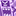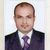# QlikView App Dev

Discussion Board for collaboration related to QlikView App Development.

cancel
Showing results for
Did you mean:Contributor III

## Aggregating data using preceding load.

Hi,

Using my first set of QVDs I want to tranform some data and load this at an aggregated level.

My first set of QVDs is at a very low granularity, I have few flags derived at that granularity but would need the data only for flag =1 .

Eg:

QVD at detailed level

Month   Account Num  Flag Amount

1             xx                      1            10

1             yy                      0             20

Summary QVD (where flag =1 )

Month            Amount

1                         10

Can I do a preceding load where I have only the data for flag = 1?

Thanks.

1 Solution

Accepted SolutionsMVP

You can also do it like

LOAD Month, Sum(If(Flag=1,Amount)) as AmountFlag1, Sum(If(Flag=0,Amount)) as AmountFlag0

FROM YourQVD (qvd)

GROUP BY Month;

6 RepliesMVP

May be like this

Amount

Where Flag = 1;

AccountNum,

Flag,

Amount

FROM ...MVP

Try

FROM YourQVD (qvd)

WHERE Flag = 1

GROUP BY Month;MVP

In summary qvd why not

Noconcatenate

Load * Resident DetailQvd Where Flag=1;Contributor III
Author

Thank you all.

I realised that there are more measures, some measures I need to bring it for both flag 0 and 1 and some with flag 1.

By using the where flag = 1 wouldnt work in this case.

Whats the best approach at the script level?

Is it a case of doing 2 loads one for flag = 1 and one without and concatenate?MVP

You can also do it like

LOAD Month, Sum(If(Flag=1,Amount)) as AmountFlag1, Sum(If(Flag=0,Amount)) as AmountFlag0

FROM YourQVD (qvd)

GROUP BY Month;Contributor III
Author

thanks so much Stefan and others.